In this post, we will be covering the implementation of a Data Pipeline in pandas for the Movelens ml-25m dataset1. The article will be divided into the following sections:

## What are Data Pipelines, and Why should we use them?

In this context, a pipeline is a software architecture that consists of executing different processing steps in sequence, on a collection of data. Each processing step can be termed as a “stage” in the pipeline. Normally, these stages are used to transform raw input data into a form that can be used by an application. Let’s illustrate this idea below: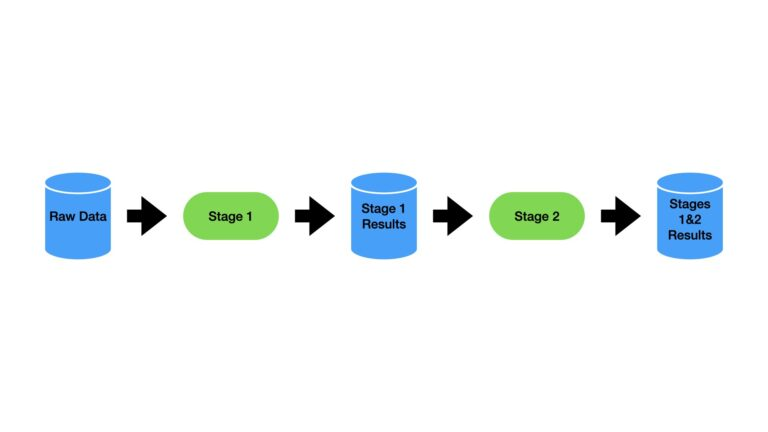A pipeline with 2 stages is depicted in the figure above. Raw input data enters the pipeline at Stage 1, where it is transformed to yield the Stage 1 Results dataset. This is subsequently fed into Stage 2, where further processing takes place. The end result are the data contained in Stages 1&2 Results.

Structuring our code into pipelines helps to facilitate many benefits. These consist of:

• Modular Code: each stage can be designed to operate independently and to fulfil a specific task. This helps to create a reusable code
• Understanding the Code: looking at the pipeline will quickly reveal what actions are being done by the software, and in what order. This helps with quickly attaining a holistic picture of what the code does
• Enhance Automation: a pipeline is an ideal structure for organising a production quality code-base for ETL (Extraction-Transform-Load) operations. The expectation is that the system will operate independently over some extended period of time
• Facilitate Scalability: stages can be added or removed as is needed by the application

Now let’s make this all tangible, by working with an example in Python.

## Create a Data Pipeline for the MovieLens ml-25m Dataset

We will work through the construction of a preprocessing data pipeline in pandas. The objective of this pipeline will be to build features out of the raw input data contained in the ml-25m dataset. The intent is that these features could be used in a movie recommender system.

### Description of the Data

These data describe 5-star rating, and free-text tagging activity, from Movelens, a movie recommendation service. Included are 25000095 ratings and 1093360 tag applications across 62423 movies. These data were created between January 9 1995 to November 21 2019, by 162541 users.

The data are contained in 6 separate csv files. Some descriptive information regarding these files, provided by the authors of the dataset1, include:

• ratings.csv : All ratings are contained within this file. Each line of this file represents one rating of one movie by one user, and has the following format: userId,movieId,rating,timestamp. Ratings are made on a 5-star scale, with half-star increments (0.5 stars – 5.0 stars).
• tags.csv : All tags are contained within this file. Each line of this file represents one tag applied to one movie by one user, and has the following format: userId,movieId,tag,timestamp. Tags are user-generated metadata about movies. Each tag is typically a single word or short phrase.
• movies.csv : Movie information is contained within this file. Each line of this file represents one movie, and has the following format: movieId,title,genres.
• links.csv : Identifiers that can be used to link to other sources of movie data are contained in this file. Each line of this file represents one movie, and has the following format: movieId,imdbId,tmdbId.
• genome-scores.csv and genome-tags.csv : The tag genome is a data structure that contains tag relevance scores for movies. The structure is a dense matrix: each movie in the genome has a value for every tag in the genome.

As I will only be using data contained within these files, I will not need to make use of links.csv. The authors also provide information regarding the id’s that can be used to match the various files together:

• User Ids : MovieLens users were selected at random for inclusion. Their ids have been anonymized. User ids are consistent between ‘ratings.csv’ and ‘tags.csv’ (i.e., the same id refers to the same user across the two files).
• Movie Ids : Only movies with at least one rating or tag are included in the dataset. Movie ids are consistent between ‘ratings.csv’, ‘tags.csv’, ‘movies.csv’, and ‘links.csv’ (i.e., the same id refers to the same movie across these four data files).

Note that Tag Ids are also present, which will allow us to match the various files associated with tag information.

Now we can make the necessary imports, and load in our data into pandas:

## imports ##
import numpy as np
import pandas as pd
from itertools import chain
import matplotlib.pyplot as plt
from typing import Dict

dfGtags   = pd.read_csv('./ml-25m/genome-tags.csv')

Okay, we’ve got our raw data into 5 separate pandas dataframes. Since I won’t be considering any temporal dependences here, let’s go ahead and remove the timestamps:

# remove timestamps
dfRatings.drop(['timestamp'],axis=1,inplace=True)
dfTags.drop(['timestamp'],axis=1,inplace=True)

### Check Ratings DataFrame

In this subsection I will examine the contents of the ratings dataframe. At the end of the subsection, I will encapsulate any actions that need to be taken solely on this dataframe into a single function. It is the intention that this function can be used as a stage in our pipeline.

First I will quickly view some of the contents of the dataframe. Secondly, I will produce a plot showing how the mean and median ratings per movie are distributed.

# initial view of ratings dataframe
dfRatings.head(5)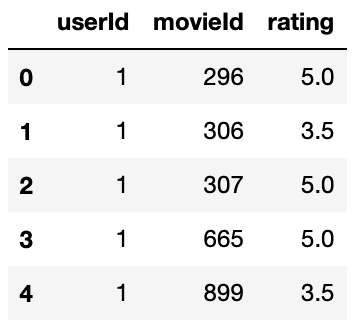# what is the mean/median distribution of movie ratings?
sMean = dfRatings.groupby(by=['movieId'])['rating'].mean().sort_values(ascending=False)
sMedian = dfRatings.groupby(by=['movieId'])['rating'].median().sort_values(ascending=False)
plt.subplots(figsize=(10, 8))
plt.plot(sMean.values,label='mean')
plt.plot(sMedian.values,label='median')
plt.xlabel('movie')
plt.ylabel('rating')
plt.title('Mean & Median Rating per Movie')
plt.legend()
plt.show()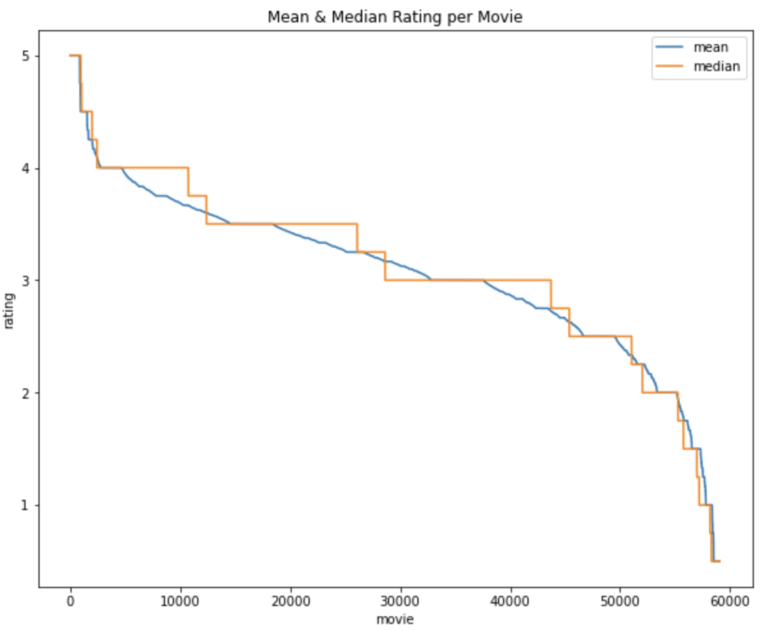# compute the number of mean/median ratings >= 4.0
print('Percentage of mean ratings greater than or equal to 4.0: {}'.format(sMean[sMean >= 4.0].shape/sMean.shape))
print('Percentage of median ratings greater than or equal to 4.0: {}'.format(sMedian[sMedian >= 4.0].shape/sMedian.shape))
Percentage of mean ratings greater than or equal to 4.0: 0.07954680170034041
Percentage of median ratings greater than or equal to 4.0: 0.18171964706081595

The plot shows how ratings are distributed over the range of movies we have in the data. Movies are sorted in descending order, as such the labels on the x-axis are a count on the number of movies in the dataset. The y-axis is the mean/median rating for each movie. It’s apparent that only a minority of the movies in our data are typically rated very highly (>= 4.0). The percentage mean rating greater than or equal to 4.0 is approximately 8%, whereas the percentage median rating greater than or equal to 4.0 is 18%.

The only preparation done directly on this dataframe is to remove the timestamp. Let’s package this into a single function:

def prepare_ratings(dfRatings: pd.DataFrame) -> pd.DataFrame:
"""
function to preprocess ratings dataframe

Inputs:
dfRatings -> dataframe containing ratings information

Outputs:
ratings dataframe without timestamp column
"""
dfRatings.drop(['timestamp'],axis=1,inplace=True)
return dfRatings

### Check Tags DataFrames

In this subsection, I will examine the contents of three dataframes associated with tags applied by users to movies. These dataframes are dfTags, dfGtags, and dfGscores. At the end of the subsection, I will encapsulate all actions that involve these dataframes into a single function. It is the intention that this function can be used as a stage in our pipeline.

First I will quickly view some of the contents from each dataframe.

# initial view of tags dataframe
dfTags.head(5)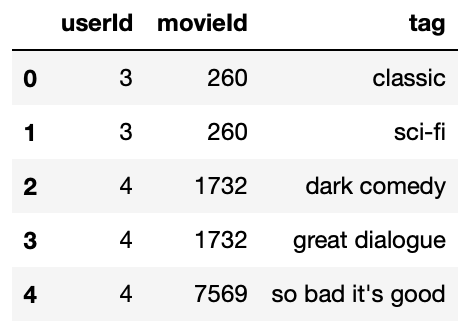# initial view of genome tags dataframe
dfGtags.head(5)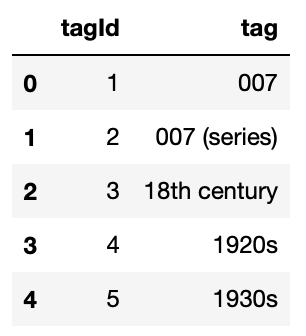# initial view of genome scores dataframe
dfGscores.head(5)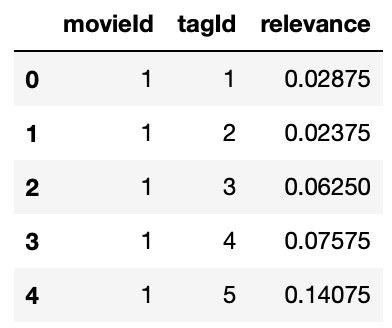We can see that these dataframes can be joined by using the tag and tagId columns. Since tag contains string values, let’s set these columns to lower case, and then proceed with the merger:

## initial preparation ##

# set tags to lower case
dfTags['tag'] = dfTags.tag.str.lower()
dfGtags['tag'] = dfGtags.tag.str.lower()

# join dfTags, dfGtags, & dfGscores
dfTagScores = pd.merge(dfTags,dfGtags,on='tag')
dfTagScores = pd.merge(dfTagScores,dfGscores,on=['movieId','tagId'])

Let’s check out how popular different tags are? Initially, I’ll do this by grouping on tagId and then count the number of tags:

# what is the frequency of tags?
sPlot = dfTagScores.groupby(by=['tagId'])['tag'].count().sort_values(ascending=False)
plt.subplots(figsize=(10, 8))
plt.plot(sPlot.values)
plt.xlabel('tag')
plt.ylabel('tag frequency')
plt.title('Tag frequency per Tag')
plt.show()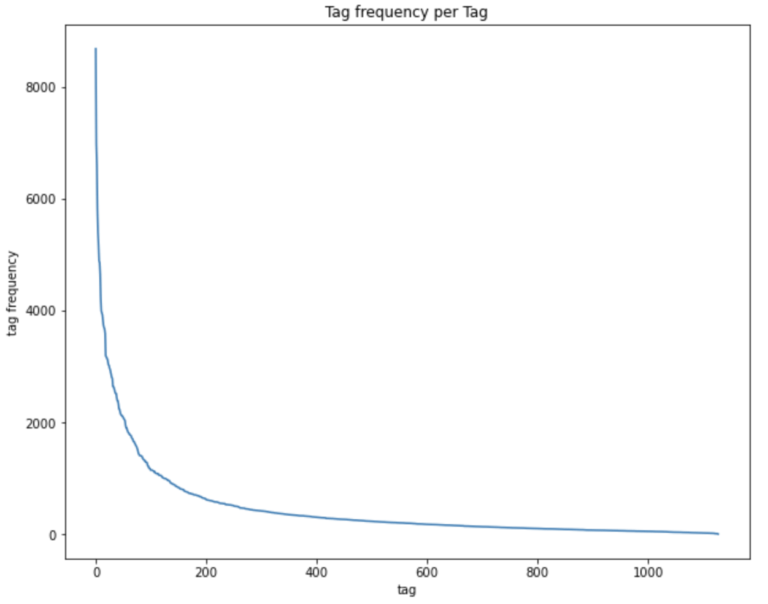We can see there’s a large spread in terms of tag frequencies. The most common tags peak at over 8000, and then rapidly fall until they level off around 1000.

However, what we really want to know is what are the most common tags used across various userId‘s? I’ll achieve this by counting the number of times unique userId’s have used a specific tagId:

# what is the frequeny of tags being used across different userId's?
dfPlot = dfTagScores[['userId','tagId']].copy()
dfPlot.drop_duplicates(inplace=True)
dfPlot['occurance'] = 1
sPlot = dfPlot.groupby(by=['tagId'])['occurance'].sum().sort_values(ascending=False)
plt.subplots(figsize=(10, 8))
plt.plot(sPlot.values)
plt.xlabel('userId/tagId pair')
plt.ylabel('tag frequency')
plt.title('Tag frequency per User-Tag pair')
plt.show()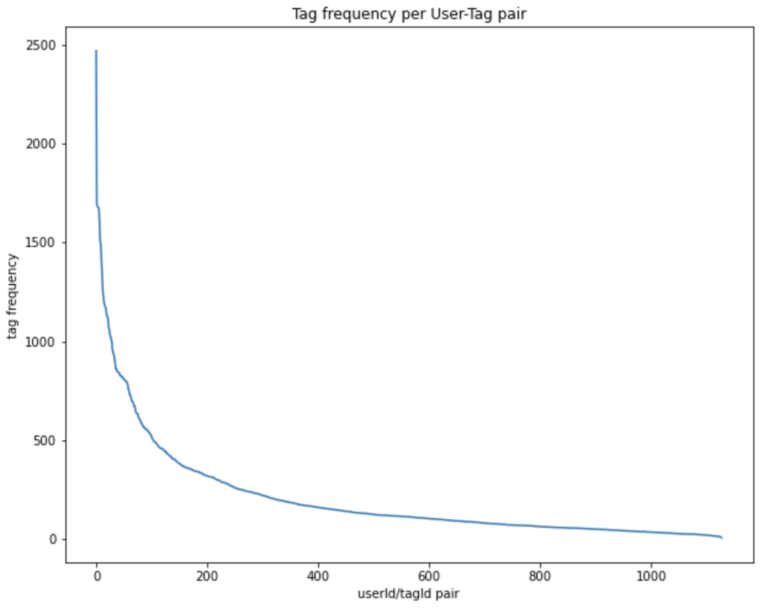This figure follows a similar form to the previous, although the scale of the vertical axis is reduced. We can see only the first ~200 tags are popularly used among different users. After this point, the graph begins to flatten out. Hence we’ll limit our analysis to only these tags.

# set cutoff threshold
threshold = 200

# extract usable tagId's
tagIds = sPlot[:threshold].index

Finally, we want to transform the tags into features we can use for machine learning. We’ll use one-hot-encoding, and then multiply each column by the associated tag relevance. Let’s do this, and then view the final result:

# OHE the tags, then multiply in the relevance
sTags = dfTagScores[dfTagScores.tagId.isin(tagIds)].tag
dfOHE = pd.get_dummies(sTags)
dfTagsOHE = dfOHE.mul(dfTagScores.relevance,axis=0)

# do final assembly of tags dataframe
dfTags = pd.concat([dfTagScores[['userId','movieId','tagId']],dfTagsOHE],axis=1)

# view the final result
dfTags.head(5)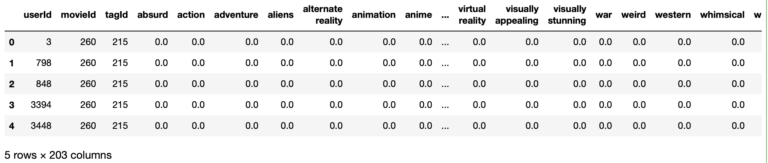Okay, these are all the steps we want to take here. Let’s now encapsulate all the operations needed to generate these tag features into a single function:

def prepare_tags(dfRatings: pd.DataFrame,
dfTags: pd.DataFrame,
dfGtags: pd.DataFrame,
dfGscores: pd.DataFrame,
threshold: float) -> pd.DataFrame:
"""
function to execute preprocessing on the tags dataframes

Inputs:
dfRatings -> dataframe containing ratings information
dfTags    -> dataframe containing tags information
dfGtags   -> dataframe containing tag genome information
dfGscores -> dataframe containing tag relevance information
thresold  -> cutoff threshold based upon tag popularity

Output:
dataframe containing the prepared tags features merged to dfRatings
"""
# drop timestamp column
dfTags.drop(['timestamp'],axis=1,inplace=True)

# set tags to lower case
dfTags['tag'] = dfTags.tag.str.lower()
dfGtags['tag'] = dfGtags.tag.str.lower()

# join dfTags, dfGtags, & dfGscores
dfTagScores = pd.merge(dfTags,dfGtags,on='tag')
dfTagScores = pd.merge(dfTagScores,dfGscores,on=['movieId','tagId'])

# extract usable tagId's based on cutoff threshold
dfTagIds = dfTagScores[['userId','tagId']].copy()
dfTagIds.drop_duplicates(inplace=True)
dfTagIds['occurance'] = 1
sTagIds = dfTagIds.groupby(by=['tagId'])['occurance'].sum().sort_values(ascending=False)
tagIds = sTagIds[:threshold].index

# OHE the tags, then multiply in the relevance
sTags = dfTagScores[dfTagScores.tagId.isin(tagIds)].tag
dfOHE = pd.get_dummies(sTags)
dfTagsOHE = dfOHE.mul(dfTagScores.relevance,axis=0)

# do final assembly of tags dataframe
dfTags = pd.concat([dfTagScores[['userId','movieId','tagId']],dfTagsOHE],axis=1)

# return merged results
return pd.merge(dfRatings,dfTags,on=['userId','movieId'])

### Check Movie DataFrame

In this subsection I will consider the last dataframe for our analysis, which contains movie information. Like before, let’s start by taking a quick view of the dataframe contents:

# initial view of movies dataframe
dfMovies.head(5)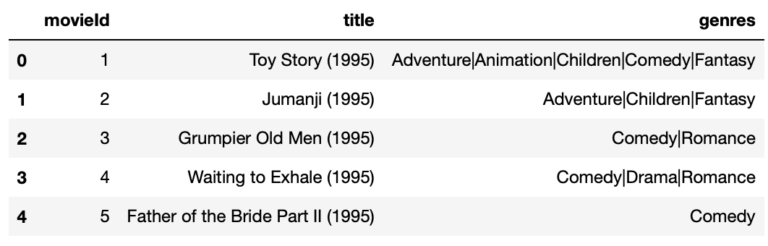The genres that apply to each movie are contained under the genres column. I would like to unpack the information in this column. Let’s build features such that each possible genre has its own unique column that can be encoded with ‘1’ if the genre applies, and otherwise ‘0’.

# obtain the unique set of genres
raw_genres = dfMovies.genres.unique()
genres     = [g.split('|') for g in raw_genres]
genres     = list(set(chain(*genres)))

# helper function for use when creating genre features
def flag_genre(row):
applicable_genres = row['genres'].split('|')
for genre in applicable_genres:
row[genre] = 1
return row

# create a set of binary features for each genre
dfGenres = pd.DataFrame(0,columns=genres,index=np.arange(dfMovies.shape))
dfMovies = pd.concat([dfMovies, dfGenres], axis=1, join='inner')
dfMovies = dfMovies.apply(flag_genre, axis=1)

We can view the results:

# view the result
dfMovies.head(5)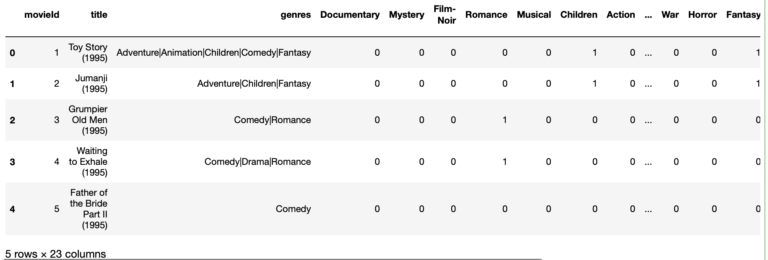These results look good. Our last processing steps will consist of:

• Removing unnecessary columns
• Joining this results with the other processed dataframes already covered
• To make the contents of the genres columns more informative per each user, let’s multiply the movie rating through all the genres
#drop irrelevant columns
dfMovies.drop(['title','genres'],axis=1,inplace=True)

#final merge
dfPrepared1 = pd.merge(dfRatings,dfTags,on=['userId','movieId'])
dfPrepared1 = pd.merge(dfPrepared1,dfMovies,on='movieId')

# multiply ratings column into movie genres
dfPrepared1.loc[:,genres] = dfPrepared1[genres].mul(dfPrepared1.rating,axis=0)

# view our final product
dfPrepared1.head(5)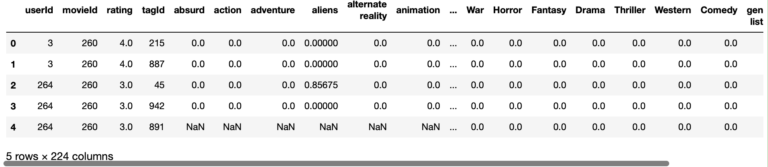Great, this concludes the steps we’ll take to build features from the movies dataframe. As before, let’s package everything into a single function:

def prepare_movies(dfRatings: pd.DataFrame,
dfMovies: pd.DataFrame) -> pd.DataFrame:
"""
function to execute preprocessing on the movies dataframe

Inputs:
dfRatings -> dataframe containing ratings information
dfMovies  -> dataframe containing movies-genre information

Output:
dataframe containing the prepared movies-genre features merged to dfRatings
"""
# helper function for use when creating genre features
def flag_genre(row):
applicable_genres = row['genres'].split('|')
for genre in applicable_genres:
row[genre] = 1
return row

# obtain the unique set of genres
raw_genres = dfMovies.genres.unique()
genres     = [g.split('|') for g in raw_genres]
genres     = list(set(chain(*genres)))

# create a set of binary features for each genre
dfGenres = pd.DataFrame(0,columns=genres,index=np.arange(dfMovies.shape))
dfMovies = pd.concat([dfMovies, dfGenres], axis=1, join='inner')
dfMovies = dfMovies.apply(flag_genre, axis=1)

#drop irrelevant columns
dfMovies.drop(['title','genres'],axis=1,inplace=True)

# merge and multiply through the ratings score
dfOut               = pd.merge(dfRatings,dfMovies,on='movieId')
dfOut.loc[:,genres] = dfOut[genres].mul(dfOut.rating,axis=0)

# return
return dfOut

### Package Everything into a Pipeline

We’ve shown how to build features in the previous subsections from the MovieLens ml-25m dataset. However, in a production environment, we will ideally not want to run isolated segments of code. Instead, let’s make use of the pandas pipe function, to string together the different functions we created already. These functions will be the different stages in our pipeline. The pipeline will carry out all the preprocessing in a single step:

# load in data

# set cutoff threshold
threshold = 200

# construct a pipeline to carry out the preprocessing work outlined previously
dfPrepared2 = dfRatings.pipe(prepare_ratings) \
.pipe(prepare_tags,dfTags=dfTags,dfGtags=dfGtags,dfGscores=dfGscores,threshold=threshold) \
.pipe(prepare_movies,dfMovies=dfMovies)

# validate that our output is the same as before
dfPrepared2.equals(dfPrepared1)
True

We can see here that the dataframe produced from the pipeline (dfPrepared2) is the same as the one we generated manually (dfPrepared1). However, the code used with the pipeline construction is far easier to read holistically. We can easily see which actions are being carried out, and in which order. This code is also far more modular, and scalable. Adding or removing features equates to adding or removing stages to the pipeline.

## Final Remarks

• What is a Data Pipeline, and why they are helpful in production software
• How to build different stages of a pipeline, using the MovieLens ml-25m dataset as an example
• How to implement a preprocessing data pipeline in Pandas

I hope you enjoyed this article, and gained some value from it.  If you would like to take a closer look at the code presented here, please take a look at my GitHub. If you have any questions or suggestions, please feel free to add a comment below. Your input is greatly appreciated.

## References

1. F. Maxwell Harper and Joseph A. Konstan. 2015. The MovieLens Datasets: History and Context. ACM Transactions on Interactive Intelligent Systems (TiiS) 5, 4: 19:1–19:19. https://doi.org/10.1145/2827872
Article Rating
Subscribe
Notify ofInline Feedbacks